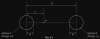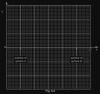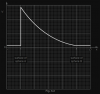# Physics: Post your doubts here!

#### ThePacifics

FREE HELP PROVIDED
Hi sorry to be a bother but if anybody needs help with any physics or maths topic im giving free help be it past paper questions or conceptual. Just email me on [email protected] and i'll reply asap good luck for exams yall

•Azzbentolo

#### tabdeelisupporter

Hi. Can someone help me with syllabus content of A Level Physics Chapter 2, Measurement Techniques.
Do we have to learn using calipers and micrometer?
And do we have to learn all techniques of measuring mass, temperature, time, etc? How much detail is required? And is the detail in Hodder book irrelevant?

#### fdshfdahda

hi. um could i get some help with June 2011 Paper 23 Q 4c ?

#### PlanetMaster

hi. um could i get some help with June 2011 Paper 23 Q 4c ?
Question Paper: https://papers.xtremepape.rs/CAIE/AS and A Level/Physics (9702)/9702_s11_qp_23.pdf

Since all the energy is converted to kinetic, we have
$$W=\frac{1}{2}mv^{2}$$
$$0.81=\frac{1}{2}mv^{2}$$
$$v=8.05ms^{-1}$$

$$\text{speed }v_{2}=8.05\times2=16.1ms^{-1}$$
Therefore, $$W=3.24J$$
Now for compression, we can use
$$W=\frac{1}{2}kx^{2}$$
and with W=3.24J, we get $$x=72mm$$

At maximum height. all KE would be converted to GPE, so
$$\frac{\frac{1}{2}k{x_{1}}^{2}}{\frac{1}{2}k{x_{2}}^{2}}=\frac{36^{2}}{72^{2}}=\frac{1}{4}$$

Hope this helps!

Last edited:

#### Himani

State
(i) what is meant by the electric potential at a point,
(ii) the relationship between electric potential at a point and electric field strength at the
point.

(b) Two similar solid metal spheres A and B, each of radius R, are situated in a vacuum such that
the separation of their centres is D, as shown in Fig. 6.1.The charge +Q on sphere A is larger than the charge +q on sphere B.

A movable point P is located on the line joining the centres of the two spheres.
The point P is a distance x from the centre of sphere A.

On Fig. 6.2, sketch a graph to show the variation with x of the electric potential V between the
centres of the two spheres.Last edited by a moderator:

#### PlanetMaster

The work per unit of charge required to move a charge from a reference point to a specified point, measured in joules per coulomb or volts.
$$\text{The electric field}=-\text{ potential gradient}$$
It comes from expressing energy changes in two different ways.
You can say work done = force x distance = qE x distance
However you can also say the work done = change in potential x charge moved = qVThe region left of Sphere A and right of Sphere B is inside the sphere so there is some potential there i.e. straight lines. The rest should be self explanatory.

Hope this helps!

#### fdshfdahda

Question Paper: https://papers.xtremepape.rs/CAIE/AS and A Level/Physics (9702)/9702_s11_qp_23.pdf

Since all the energy is converted to kinetic, we have
$$W=\frac{1}{2}mv^{2}$$
$$0.81=\frac{1}{2}mv^{2}$$
$$v=8.05ms^{-1}$$

$$\text{speed }v_{2}=8.05\times2=16.1ms^{-1}$$
Therefore, $$W=3.24J$$
Now for compression, we can use
$$W=\frac{1}{2}kx^{2}$$
and with W=3.24J, we get $$x=72mm$$

At maximum height. all KE would be converted to GPE, so
$$\frac{\frac{1}{2}k{x_{1}}^{2}}{\frac{1}{2}k{x_{2}}^{2}}=\frac{36^{2}}{72^{2}}=\frac{1}{4}$$

Hope this helps!
thank you so much•PlanetMaster

#### PlanetMaster

From a velocity-time graph, the acceleration at time t is given by the gradient at time t. This gives the instantaneous acceleration at that time.
In this graph, the gradient and hence the acceleration is changing.

Note that the gradient at time t = 3s is obtained by drawing a tangent at that point and calculating its gradient. We cannot just take the coordinate at that point (3, 6) to obtain acceleration (as done for choice D).

A tangent at time t = 3s would pass almost at/close to points (0, 2) and (6, 10).
$$\text{Gradient}=\frac{(10–2)}{(6 – 0)}=\frac{8}{6}=1.33ms^{-1}$$

P.S. This is taken from an earlier post

#### [email protected]

From a velocity-time graph, the acceleration at time t is given by the gradient at time t. This gives the instantaneous acceleration at that time.
In this graph, the gradient and hence the acceleration is changing.

Note that the gradient at time t = 3s is obtained by drawing a tangent at that point and calculating its gradient. We cannot just take the coordinate at that point (3, 6) to obtain acceleration (as done for choice D).

A tangent at time t = 3s would pass almost at/close to points (0, 2) and (6, 10).
$$\text{Gradient}=\frac{(10–2)}{(6 – 0)}=\frac{8}{6}=1.33ms^{-1}$$

P.S. This is taken from an earlier post
Thank you

•PlanetMaster

#### Kar345

How did Physics Paper 42 go???

#### PlanetMaster

View attachment 65045
so i solved this and got B as the answer but ms says the right answer is 8cm/s. Can anyone please help me with this?Since the collision is perfectly elastic, we can use
$$v_{1}i+v_{1}f=v_{2}i+v_{2}f$$ (You might have a different variant of this formula depending on your board)

Substituting in our values, we get
$$6+2=0+v_{2}f$$

And therefore,
$$v_{2}f=8$$

Hope this helps!

•fdshfdahda

#### fdshfdahda

Since the collision is perfectly elastic, we can use
$$v_{1}i+v_{1}f=v_{2}i+v_{2}f$$ (You might have a different variant of this formula depending on your board)

Substituting in our values, we get
$$6+2=0+v_{2}f$$

And therefore,
$$v_{2}f=8$$

Hope this helps!
i thought the formua was mu1+mu2=mv1+mv2? and if the directions were opposite do we have to change the signs?

#### PlanetMaster

i thought the formua was mu1+mu2=mv1+mv2? and if the directions were opposite do we have to change the signs?
The formula I used earlier is derived from this under special conditions for elastic collisions.
Since velocity is a vector quantity, the signs need to be changed with directions.

View attachment 65046
Is the 6.3 s for the whole graph or just beween the pulses? i attempted this and got 2.1. The answer should actually be C.
The pulse sent by transmitter is reflected and returns to the transmitter so it takes it $$6.3\mu s\times2$$ to travel from the transmitter to the reflector and back to transmitter. So we'll need a time-base setting of $$12.6\mu s/3cm=4.2\mu s\ cm^{-1}$$.

Im sorry for asking a lot xD My cie is within two weeks so help would be appreciatedYou can never ask too many questions. Keep em coming.. (terms and conditions apply!)

#### [email protected]

How to solve this?

A moving body undergoes uniform acceleration while travelling in a straight line between points X, Y and Z. The distances XY and YZ are both 40 m. The time to travel from X to Y is 12 s and from Y to Z is 6.0 s.

What is the acceleration of the body?

A) $$0.37 m s^{–2}$$
B) $$0.49 m s^{–2}$$
C) $$0.56 m s^{–2}$$
D) $$1.1 m s^{–2}$$

Last edited by a moderator:

#### PlanetMaster

How to solve this?

A moving body undergoes uniform acceleration while travelling in a straight line between points X, Y and Z. The distances XY and YZ are both 40 m. The time to travel from X to Y is 12 s and from Y to Z is 6.0 s.

What is the acceleration of the body?

A) $$0.37 m s^{–2}$$
B) $$0.49 m s^{–2}$$
C) $$0.56 m s^{–2}$$
D) $$1.1 m s^{–2}$$
There are two unknowns here, starting speed $$u$$ and acceleration $$a$$. Using $$s=ut+\frac{1}{2}at^2$$, we’re given that in 12 seconds from X to Y:
$$\qquad 40=12u+\frac{1}{2}12^2a=12u+72a$$
and in 18 seconds from X to Z:
$$\qquad 80=18u+\frac{1}{2}18^2a=18u+162a$$
Multiplying the first equation by $$\frac{3}{2}$$ we get:
$$\qquad 60=18u+108a$$
and subtracting this from the second equation gives:
$$\qquad 20=54a$$
Therefore the answer is that the acceleration $$a=\frac{10}{27}$$ m/s$$^2$$. We’re not asked about $$u$$ so we needn’t calculate it.

#### [email protected]

There are two unknowns here, starting speed $$u$$ and acceleration $$a$$. Using $$s=ut+\frac{1}{2}at^2$$, we’re given that in 12 seconds from X to Y:
$$\qquad 40=12u+\frac{1}{2}12^2a=12u+72a$$
and in 18 seconds from X to Z:
$$\qquad 80=18u+\frac{1}{2}18^2a=18u+162a$$
Multiplying the first equation by $$\frac{3}{2}$$ we get:
$$\qquad 60=18u+108a$$
and subtracting this from the second equation gives:
$$\qquad 20=54a$$
Therefore the answer is that the acceleration $$a=\frac{10}{27}$$ m/s$$^2$$. We’re not asked about $$u$$ so we needn’t calculate it.
Thankyou•PlanetMaster